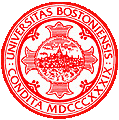# Programming Assignment 4

CS 585 HW 4
Kaiyuan Fan
kaikang Zhu
10/31/2018

## Problem Definition

The problem of this assignment is divided into two parts. First part is segmentation of hands, the second part is tracking two datasets.

1. Part 1: Segmentation of hands. In this part, you are given a sequence of video frames in which a person is playing the piano with both hands. Try to develop an algorithm to identify the pianist's hands. Portions of the hands are sometimes in deep shadow, which creates a challenging imaging situation.
2. Part 2: Tracking. The goal of this part of the programming assignment is for you to learn more about the practical issues that arise when designing a tracking system. You are asked to track moving objects in video sequences, i.e., identifying the same object from frame to frame.

## Method and Implementation

1. For part 1:To obtain the segmentation of hands, we can calculate the difference between two frames because hands are the only object move in images. I first turn two images into grayscale and use absdiff() to calculate the difference.
2. Then use blur() to smooth the difference and threshold the difference to make it a binary image. After that, use connectedComponentsWithStats() to label connected areas in the difference. Besides the background, I assume that biggest connected area is the pianist's hands. We can obtain the centroids of areas using the previous function. Use the centroid to draw the bounding box. The upper box is the right hand and the lower box is the left hand.

1. For Part2 :In bat datasets, we are given centroids of detection at each frame. We are considering two frames at a time.
2. For all bats in the current frame, find a distance to the prediction from the previous frame. Predictions are created by the AlphaBetaFilter. We are using a greedy matching method find the least distance to match the current frame object to previous frame object.
3. We are setting a tolerance error value if the least distance exceeds the tolerance, the match failed, the object in the current frame consider a new object. If there are any objects in the previous frame didn't get matched, we consider the object left the scene.

Part1 Functions: void double_threshold(cv::Mat& img, cv::Mat& dst, double thresh_1, double thresh_2);//use threshold to binary the image vector<pair<int, int>> get_n4(int c_row, int c_col, int n_rows, int n_cols);//return n4 neighbour of a pixel colckwise starting fomr the west Part2 Functions: vector<vector<float>> getObjects(String filename);// get objects from current frame by reading localization file vector<vector<float>> AlphaBetaFilter(vector<vector<float>> current);// predict current frame objects location at the next frame based on the velocity and previous location int greedyMatch(int a, int b, vector<vector<float>> prediction);//count objects positions in current frame with the prediction points, return the lowest point id (least distance) int handleTracks(int a, int b, int id, vector<vector<float>> prediction);// given the current object and the least distance object id, calculate the distance to handle new and old objects vector<vector<float>> MatchDrawUpdate(vector<vector<float>> current, vector<vector<float>> prediction, Mat &binary3C, vector<vector<char>> colors);//match objects in the current frame with the predications, draw lines if matched, update velocities and previous locations Mat updateMap(Mat &binary, Mat &binary3C);//update the batmap OpenCV library functions: absdiff blur connectedComponentsWithStats rectangle resize line cvtColor

## Experiments

I am applying below template to detect hand shapes.

original segmentedexamples challenging situation
Success when new objects come inFailed when object move too fast, and objects touch and leave situationBat track video

## Discussion

Results are showed as above.

How do you decide to begin new tracks and terminate old tracks as the objects enter and leave the field of view?

we are operating on the current frame of objects and try to match them with the previous frame prediction. If the object in the current frame doesn't match means it's a new object. If object in previous frame doesn't match means the object leave the field of view.

What happens with your algorithm when objects touch and occlude each other, and how could you handle this so you do not break track?

when two objects touch, our algorithm will consider one of the objects leaves the field of view, and consider the two objects as one bigger object.

What happens when there are spurious detections that do not connect with other measurements in subsequent frames?

Spurious detections happens like velocity of object is too high, will cause the track break and consider the object as different objects in the subsequent frames.

What are the advantages and drawbacks of different kinematic models: Do you need to model the velocity of the objects, or is it sufficient to just consider the distances between the objects in subsequent frames?

Having a velocity model can help us to predict the position of the object. In the tracking process, it's important because if we consider greedy matching only the two close objects in two subsequent frames can be different objects. Also, the measurement has a lot of noise.

## Conclusions

In conclusion, frame difference may not a good way to segment and track objects. It depends on the brightness and variation of each frames. We can use some other methods to improve the algorithm under different circumstances. We find segmentation and tracking are interesting topics and we can improve our algorithm by solving those challenging situations.

## Credits and Bibliography

https://docs.opencv.org/3.3.1/d3/dc0/group__imgproc__shape.html#ga107a78bf7cd25dec05fb4dfc5c9e765f accessed at 10/25/2018

https://docs.opencv.org/2.4/modules/core/doc/operations_on_arrays.html accessed at 10/25/2018